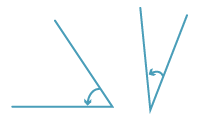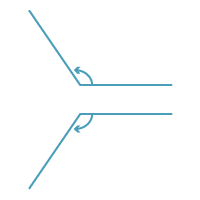Acute angle

An acute angle is smaller than a right angle. An acute angle is less than 90°. Here are two examples:Detailed description

One way to remember an acute angle is to think that cute things are usually little. Also, acute means sharp, as in sharp pain.

Obtuse angle

Obtuse angles are between a right angle and a straight angle; so an obtuse angle is larger than 90°, but smaller than 180°. Obtuse is the opposite of sharp – it means dull. Here are two examples:Detailed description

Reflex angles

Reflex angles are larger than 180°, but less than 360°. Here is one example:Detailed description

Comparing angles

We compare angles by looking to see if they are smaller or larger than the types of angles shown above, or by measuring them as shown in the next section.